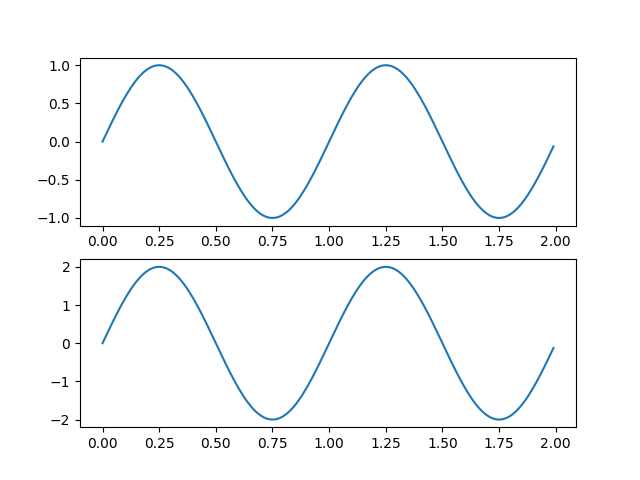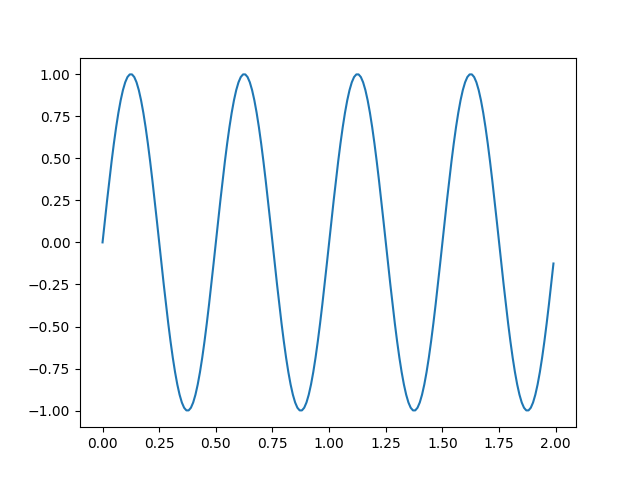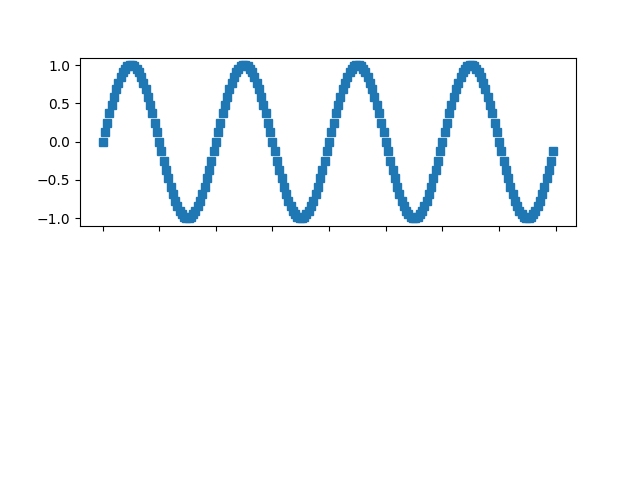Managing multiple figures in pyplot¶

matplotlib.pyplot uses the concept of a current figure and current axes. Figures are identified via a figure number that is passed to figure. The figure with the given number is set as current figure. Additionally, if no figure with the number exists, a new one is created.

Note

We discourage working with multiple figures in pyplot because managing the current figure is cumbersome and error-prone. Instead, we recommend to use the object-oriented approach and call methods on Figure and Axes instances.

import matplotlib.pyplot as plt
import numpy as np

t = np.arange(0.0, 2.0, 0.01)
s1 = np.sin(2*np.pi*t)
s2 = np.sin(4*np.pi*t)

Create figure 1Out:

[<matplotlib.lines.Line2D object at 0x7fd223470e50>]

Create figure 2Out:

[<matplotlib.lines.Line2D object at 0x7fd20151cc10>]

Now switch back to figure 1 and make some changesTotal running time of the script: ( 0 minutes 1.376 seconds)

Keywords: matplotlib code example, codex, python plot, pyplot Gallery generated by Sphinx-Gallery